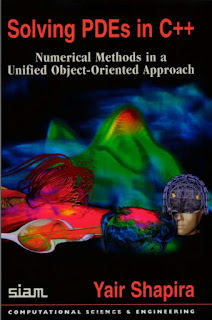C++

# Solving PDEs in C++ Numerical Methods in a Unified Object-Oriented Approach by Yair Shapira PDF Free DownloadThis comprehensive book not only introduces the C and C++ programming languages but also shows how to use them in the numerical solution of partial differential equations (PDEs). It leads the reader through the entire solution process, from the original PDE, through the discretization stage, to the numerical solution of the resulting algebraic system. The well-debugged and tested code segments implement the numerical methods efficiently and transparently. Basic and advanced numerical methods are introduced and implemented easily and efficiently in a unified object-oriented approach. It introduces a unified approach for the implementation of complex mathematical objects, such as unstructured mesh, sparse matrix, and multigrid hierarchy, often used in numerical modeling, where the high level of abstraction available in C++ is particularly useful, Included is all the required background in programming, PDEs, and numerical methods, and an elementary background in linear algebra and calculus. Useful exercises and solutions conclude each chapter.